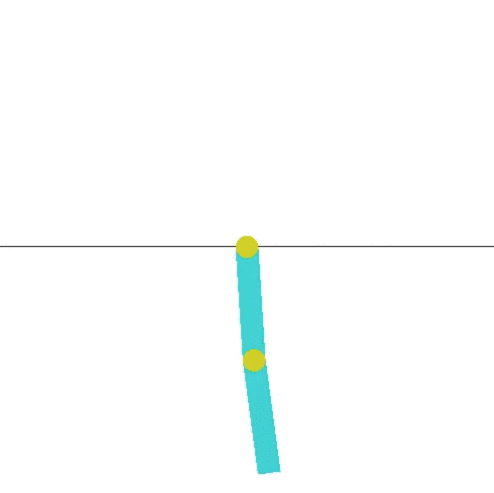• +91-9872993883
• +91-8283824812
• info@ris-ai.com

# Acrobot OpenAI Gym¶

## >>>Learn Machine learning on finger tips in limited time period *** CAll NOW (9872993883)!!!!! ***<<<

### Acrobot Python Tutorial

#### What is the main Goal of Acrobot?¶

The problem setting is to solve the Acrobot problem in OpenAI gym. The acrobot system includes two joints and two links, where the joint between the two links is actuated. Initially, the links are hanging downwards, and the goal is to swing the end of the lower link up to a given height (the black horizontal line)

##### The following diagram shows the movement of the Acrobot in a brief sequence of timesteps, from the start to an end position: ¶#### Here are the steps for the movement of the acrobot:¶

In [ ]:
import numpy as np
import gym
from keras.models import Sequential
from keras.layers import Dense, Activation, Flatten

from rl.agents.dqn import DQNAgent
from rl.policy import BoltzmannQPolicy, EpsGreedyQPolicy
from rl.memory import SequentialMemory
ENV_NAME = 'Acrobot-v1'
env = gym.make(ENV_NAME)
Using TensorFlow backend.

In [ ]:
## play for rondom action, without being trained
for i_episode in range(5):
observation = env.reset()
for t in range(100):
env.render()
print(observation)
action = env.action_space.sample()
observation, reward, done, info = env.step(action)
if done:
print("Episode finished after {} timesteps".format(t+1))
break
env.close()

In [ ]:
# Get the environment and extract the number of actions.
np.random.seed(123)
env.seed(123)
nb_actions = env.action_space.n
model = Sequential()
#
print(model.summary())

In [ ]:
# # Finally, we configure and compile our agent. You can use every built-in Keras optimizer and
# # even the metrics!
memory = SequentialMemory(limit=50000, window_length=1)
policy = EpsGreedyQPolicy()
dqn = DQNAgent(model=model, nb_actions=nb_actions, memory=memory, nb_steps_warmup=50,
target_model_update=200,train_interval=4, policy=policy)
#
# # Okay, now it's time to learn something! We visualize the training here for show, but this
# # slows down training quite a lot. You can always safely abort the training prematurely using
# # Ctrl + C.
# dqn.fit(env, nb_steps=10000, visualize=False, verbose=2)
#
# # After training is done, we save the final weights.
# dqn.save_weights('dqn_{}_weights.h5f'.format(ENV_NAME), overwrite=True)
# Finally, evaluate our algorithm for 5 episodes.
#
weights_filename = 'dqn_{}_weights.h5f'.format(ENV_NAME)
#     # if args.weights:
#     #     weights_filename = args.weights

env.close()